Domain and Range

LEARNING OBJECTIVES

By the end of this lesson, you will be able to:

• Find the domain of a function defined by an equation.
• Graph piecewise-defined functions.

If you’re in the mood for a scary movie, you may want to check out one of the five most popular horror movies of all time—I am Legend, Hannibal, The Ring, The Grudge, and The Conjuring. Figure 1 shows the amount, in dollars, each of those movies grossed when they were released as well as the ticket sales for horror movies in general by year. Notice that we can use the data to create a function of the amount each movie earned or the total ticket sales for all horror movies by year. In creating various functions using the data, we can identify different independent and dependent variables, and we can analyze the data and the functions to determine the domain and range. In this section, we will investigate methods for determining the domain and range of functions such as these.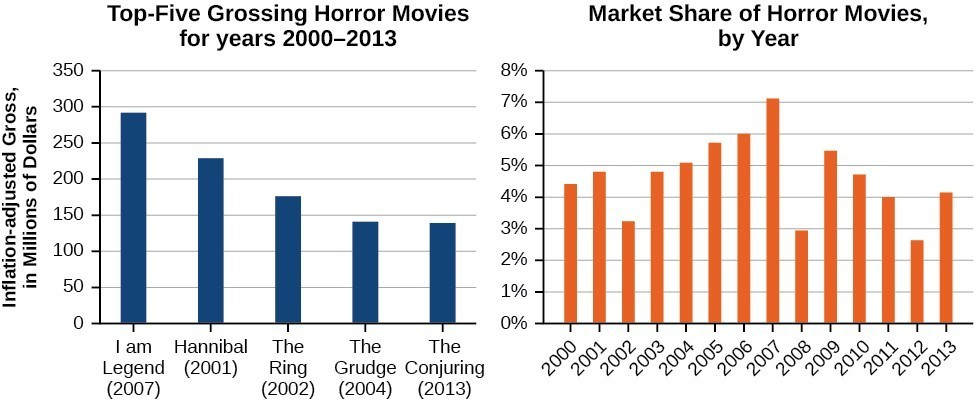Figure 1. Based on data compiled by www.the-numbers.com.

Find the domain of a function defined by an equation

In Functions and Function Notation, we were introduced to the concepts of domain and range. In this section, we will practice determining domains and ranges for specific functions. Keep in mind that, in determining domains and ranges, we need to consider what is physically possible or meaningful in real-world examples, such as tickets sales and year in the horror movie example above. We also need to consider what is mathematically permitted. For example, we cannot include any input value that leads us to take an even root of a negative number if the domain and range consist of real numbers. Or in a function expressed as a formula, we cannot include any input value in the domain that would lead us to divide by 0.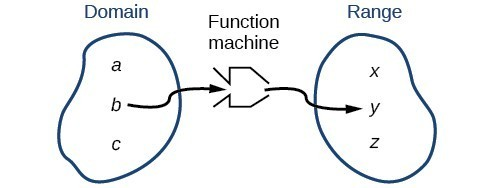Figure 2

We can visualize the domain as a “holding area” that contains “raw materials” for a “function machine” and the range as another “holding area” for the machine’s products.

We can write the domain and range in interval notation, which uses values within brackets to describe a set of numbers. In interval notation, we use a square bracket [ when the set includes the endpoint and a parenthesis ( to indicate that the endpoint is either not included or the interval is unbounded. For example, if a person has $100 to spend, he or she would need to express the interval that is more than 0 and less than or equal to 100 and write $\left(0,\text{ }100\right]$. We will discuss interval notation in greater detail later. Let’s turn our attention to finding the domain of a function whose equation is provided. Oftentimes, finding the domain of such functions involves remembering three different forms. First, if the function has no denominator or an even root, consider whether the domain could be all real numbers. Second, if there is a denominator in the function’s equation, exclude values in the domain that force the denominator to be zero. Third, if there is an even root, consider excluding values that would make the radicand negative. Before we begin, let us review the conventions of interval notation: • The smallest term from the interval is written first. • The largest term in the interval is written second, following a comma. • Parentheses, ( or ), are used to signify that an endpoint is not included, called exclusive. • Brackets, [ or ], are used to indicate that an endpoint is included, called inclusive. The table below gives a summary of interval notation.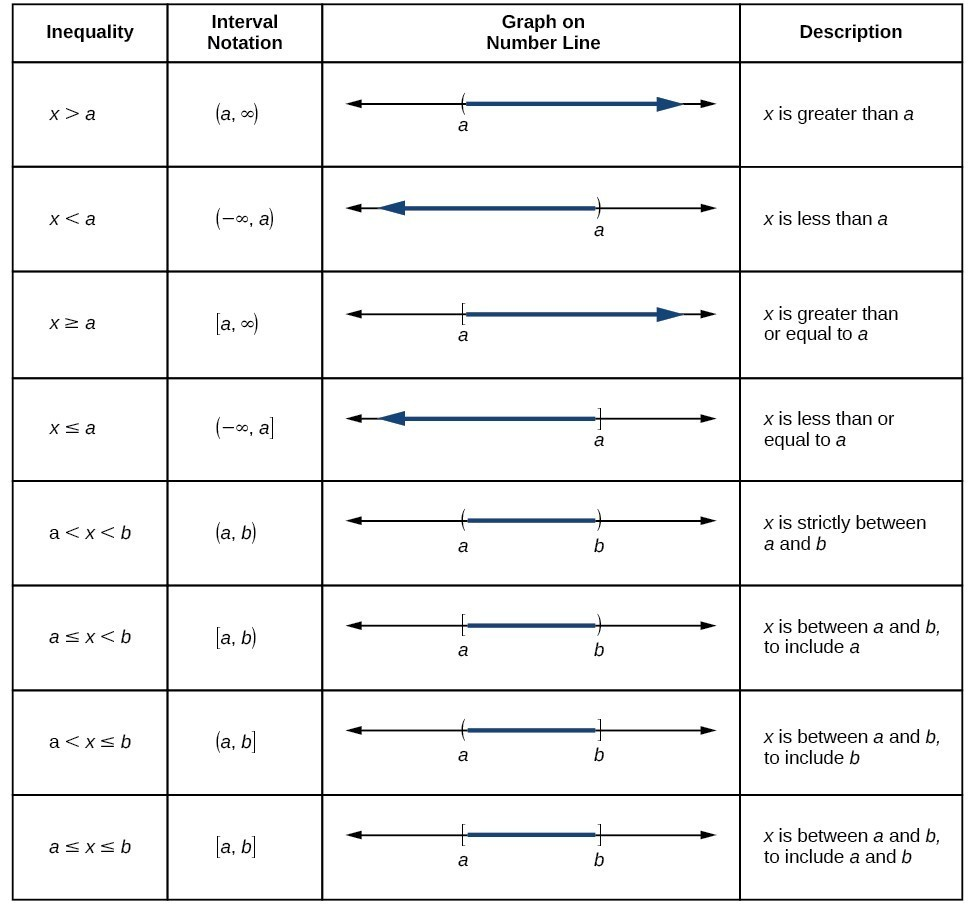Example 1: Finding the Domain of a Function as a Set of Ordered Pairs Find the domain of the following function: $\left\{\left(2,\text{ }10\right),\left(3,\text{ }10\right),\left(4,\text{ }20\right),\left(5,\text{ }30\right),\left(6,\text{ }40\right)\right\}$ . Solution First identify the input values. The input value is the first coordinate in an ordered pair. There are no restrictions, as the ordered pairs are simply listed. The domain is the set of the first coordinates of the ordered pairs. $\left\{2,3,4,5,6\right\}$ Try It 1 Find the domain of the function: $\left\{\left(-5,4\right),\left(0,0\right),\left(5,-4\right),\left(10,-8\right),\left(15,-12\right)\right\}$ Solution How To: Given a function written in equation form, find the domain. 1. Identify the input values. 2. Identify any restrictions on the input and exclude those values from the domain. 3. Write the domain in interval form, if possible. Example 2: Finding the Domain of a Function Find the domain of the function $f\left(x\right)={x}^{2}-1$. Solution The input value, shown by the variable $x$ in the equation, is squared and then the result is lowered by one. Any real number may be squared and then be lowered by one, so there are no restrictions on the domain of this function. The domain is the set of real numbers. In interval form, the domain of $f$ is $\left(-\infty ,\infty \right)$. Try It 2 Find the domain of the function: $f\left(x\right)=5-x+{x}^{3}$. Solution How To: Given a function written in an equation form that includes a fraction, find the domain. 1. Identify the input values. 2. Identify any restrictions on the input. If there is a denominator in the function’s formula, set the denominator equal to zero and solve for $x$ . If the function’s formula contains an even root, set the radicand greater than or equal to 0, and then solve. 3. Write the domain in interval form, making sure to exclude any restricted values from the domain. Example 3: Finding the Domain of a Function Involving a Denominator (Rational Function) Find the domain of the function $f\left(x\right)=\frac{x+1}{2-x}$. Solution When there is a denominator, we want to include only values of the input that do not force the denominator to be zero. So, we will set the denominator equal to 0 and solve for $x$. $\begin{cases}2-x=0\hfill \\ -x=-2\hfill \\ x=2\hfill \end{cases}$ Now, we will exclude 2 from the domain. The answers are all real numbers where $x<2$ or $x>2$. We can use a symbol known as the union, $\cup$, to combine the two sets. In interval notation, we write the solution: $\left(\mathrm{-\infty },2\right)\cup \left(2,\infty \right)$.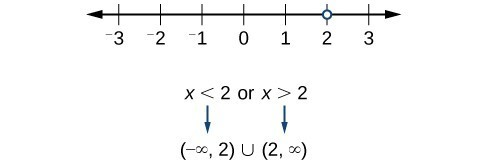Figure 3 In interval form, the domain of $f$ is $\left(-\infty ,2\right)\cup \left(2,\infty \right)$. Try It 3 Find the domain of the function: $f\left(x\right)=\frac{1+4x}{2x - 1}$. Solution How To: Given a function written in equation form including an even root, find the domain. 1. Identify the input values. 2. Since there is an even root, exclude any real numbers that result in a negative number in the radicand. Set the radicand greater than or equal to zero and solve for $x$. 3. The solution(s) are the domain of the function. If possible, write the answer in interval form. Example 4: Finding the Domain of a Function with an Even Root Find the domain of the function $f\left(x\right)=\sqrt{7-x}$. Solution When there is an even root in the formula, we exclude any real numbers that result in a negative number in the radicand. Set the radicand greater than or equal to zero and solve for $x$. $\begin{cases}7-x\ge 0\hfill \\ -x\ge -7\hfill \\ x\le 7\hfill \end{cases}$ Now, we will exclude any number greater than 7 from the domain. The answers are all real numbers less than or equal to $7$, or $\left(-\infty ,7\right]$. Try It 4 Find the domain of the function $f\left(x\right)=\sqrt{5+2x}$. Solution Q & A Can there be functions in which the domain and range do not intersect at all? Yes. For example, the function $f\left(x\right)=-\frac{1}{\sqrt{x}}$ has the set of all positive real numbers as its domain but the set of all negative real numbers as its range. As a more extreme example, a function’s inputs and outputs can be completely different categories (for example, names of weekdays as inputs and numbers as outputs, as on an attendance chart), in such cases the domain and range have no elements in common. In the previous examples, we used inequalities and lists to describe the domain of functions. We can also use inequalities, or other statements that might define sets of values or data, to describe the behavior of the variable in set-builder notation. For example, $\left\{x|10\le x<30\right\}$ describes the behavior of $x$ in set-builder notation. The braces { } are read as “the set of,” and the vertical bar | is read as “such that,” so we would read $\left\{x|10\le x<30\right\}$ as “the set of x-values such that 10 is less than or equal to $x$, and $x$ is less than 30.” The table below compares inequality notation, set-builder notation, and interval notation. Inequality Notation Set-builder Notation Interval Notation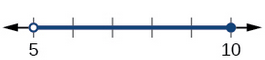5 < h ≤ 10 { h | 5 < h ≤ 10} (5, 10]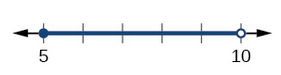5 ≤ h < 10 { h | 5 ≤ h < 10} [5, 10]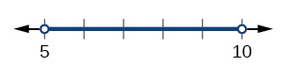5 < h < 10 { h | 5 < 10 } (5, 10)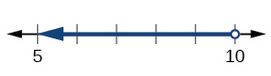h < 10 { h | h < 10 } ( −∞, 10)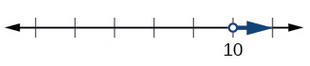h ≥ 10 { h | h ≥ 10 } [10, ∞ )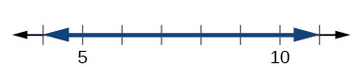All real numbers ( −∞, ∞ ) To combine two intervals using inequality notation or set-builder notation, we use the word “or.” As we saw in earlier examples, we use the union symbol, $\cup$, to combine two unconnected intervals. For example, the union of the sets $\left\{2,3,5\right\}$ and $\left\{4,6\right\}$ is the set $\left\{2,3,4,5,6\right\}$. It is the set of all elements that belong to one or the other (or both) of the original two sets. For sets with a finite number of elements like these, the elements do not have to be listed in ascending order of numerical value. If the original two sets have some elements in common, those elements should be listed only once in the union set. For sets of real numbers on intervals, another example of a union is $\left\{x|\text{ }|x|\ge 3\right\}=\left(-\infty ,-3\right]\cup \left[3,\infty \right)$ This video describes how to use interval notation to describe a set. This video describes how to use Set-Builder notation to describe a set. A General Note: Set-Builder Notation and Interval Notation Set-builder notation is a method of specifying a set of elements that satisfy a certain condition. It takes the form $\left\{x|\text{statement about }x\right\}$ which is read as, “the set of all $x$ such that the statement about $x$ is true.” For example, $\left\{x|4<x\le 12\right\}$ Interval notation is a way of describing sets that include all real numbers between a lower limit that may or may not be included and an upper limit that may or may not be included. The endpoint values are listed between brackets or parentheses. A square bracket indicates inclusion in the set, and a parenthesis indicates exclusion from the set. For example, $\left(4,12\right]$ How To: Given a line graph, describe the set of values using interval notation. 1. Identify the intervals to be included in the set by determining where the heavy line overlays the real line. 2. At the left end of each interval, use [ with each end value to be included in the set (solid dot) or ( for each excluded end value (open dot). 3. At the right end of each interval, use ] with each end value to be included in the set (filled dot) or ) for each excluded end value (open dot). 4. Use the union symbol $\cup$ to combine all intervals into one set. Example 5: Describing Sets on the Real-Number Line Describe the intervals of values shown in Figure 4 using inequality notation, set-builder notation, and interval notation.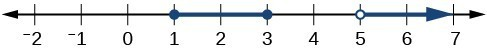Figure 4 Solution To describe the values, $x$, included in the intervals shown, we would say, ” $x$ is a real number greater than or equal to 1 and less than or equal to 3, or a real number greater than 5.”  Inequality $1\le x\le 3\text{or}x>5$ Set-builder notation $\left\{x|1\le x\le 3\text{or}x>5\right\}$ Interval notation $\left[1,3\right]\cup \left(5,\infty \right)$ Remember that, when writing or reading interval notation, using a square bracket means the boundary is included in the set. Using a parenthesis means the boundary is not included in the set. Try It 5 Given Figure 5, specify the graphed set in 1. words 2. set-builder notation 3. interval notation Solution Finding Domain and Range from Graphs Another way to identify the domain and range of functions is by using graphs. Because the domain refers to the set of possible input values, the domain of a graph consists of all the input values shown on the x-axis. The range is the set of possible output values, which are shown on the y-axis. Keep in mind that if the graph continues beyond the portion of the graph we can see, the domain and range may be greater than the visible values. See Figure 6.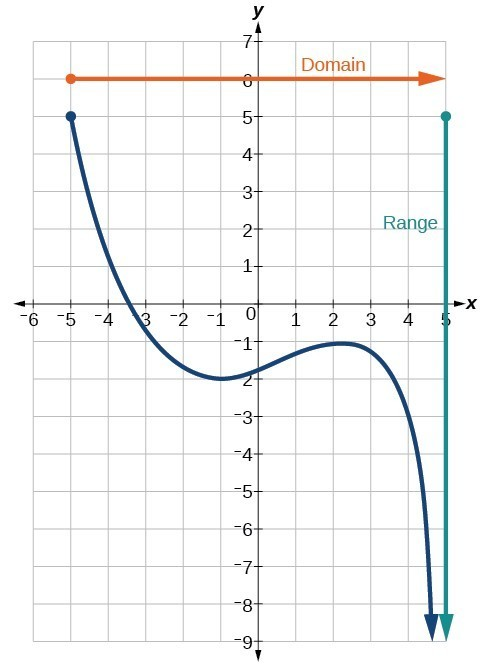Figure 6 We can observe that the graph extends horizontally from $-5$ to the right without bound, so the domain is $\left[-5,\infty \right)$. The vertical extent of the graph is all range values $5$ and below, so the range is $\left(\mathrm{-\infty },5\right]$. Note that the domain and range are always written from smaller to larger values, or from left to right for domain, and from the bottom of the graph to the top of the graph for range. Example 6: Finding Domain and Range from a Graph Find the domain and range of the function $f$ whose graph is shown in Figure 7.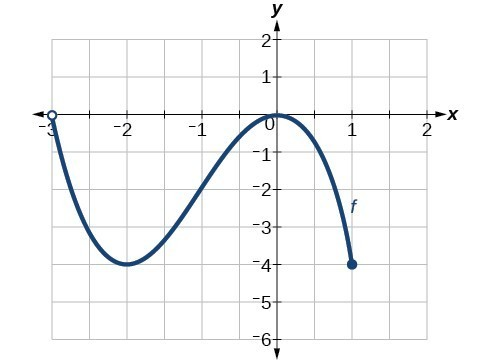Figure 7 Solution We can observe that the horizontal extent of the graph is –3 to 1, so the domain of $f$ is $\left(-3,1\right]$.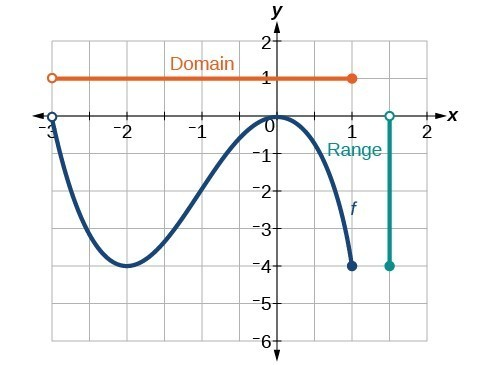Figure 8 The vertical extent of the graph is 0 to –4, so the range is $\left[-4,0\right)$. Example 7: Finding Domain and Range from a Graph of Oil Production Find the domain and range of the function $f$ whose graph is shown in Figure 9.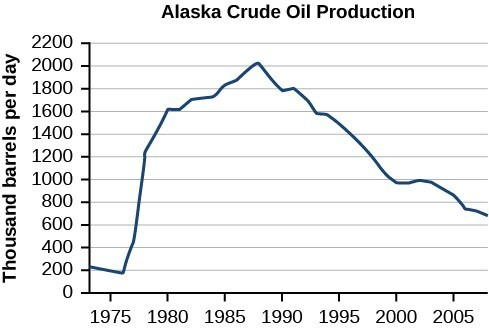Figure 9. (credit: modification of work by the U.S. Energy Information Administration) Solution The input quantity along the horizontal axis is “years,” which we represent with the variable $t$ for time. The output quantity is “thousands of barrels of oil per day,” which we represent with the variable $b$ for barrels. The graph may continue to the left and right beyond what is viewed, but based on the portion of the graph that is visible, we can determine the domain as $1973\le t\le 2008$ and the range as approximately $180\le b\le 2010$. In interval notation, the domain is [1973, 2008], and the range is about [180, 2010]. For the domain and the range, we approximate the smallest and largest values since they do not fall exactly on the grid lines. Try It 6 Given the graph in Figure 10, identify the domain and range using interval notation.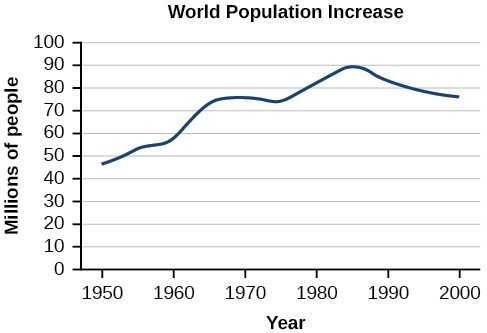Figure 10 Solution Q & A Can a function’s domain and range be the same? Yes. For example, the domain and range of the cube root function are both the set of all real numbers. Finding Domain and Range from Graphs We will now return to our set of toolkit functions to determine the domain and range of each. How To: Given the formula for a function, determine the domain and range. 1. Exclude from the domain any input values that result in division by zero. 2. Exclude from the domain any input values that have nonreal (or undefined) number outputs. 3. Use the valid input values to determine the range of the output values. 4. Look at the function graph and table values to confirm the actual function behavior. Example 8: Finding the Domain and Range Using Toolkit Functions Find the domain and range of $f\left(x\right)=2{x}^{3}-x$. Solution There are no restrictions on the domain, as any real number may be cubed and then subtracted from the result. The domain is $\left(-\infty ,\infty \right)$ and the range is also $\left(-\infty ,\infty \right)$. Example 9: Finding the Domain and Range Find the domain and range of $f\left(x\right)=\frac{2}{x+1}$. Solution We cannot evaluate the function at $-1$ because division by zero is undefined. The domain is $\left(-\infty ,-1\right)\cup \left(-1,\infty \right)$. Because the function is never zero, we exclude 0 from the range. The range is $\left(-\infty ,0\right)\cup \left(0,\infty \right)$. Example 10: Finding the Domain and Range Find the domain and range of $f\left(x\right)=2\sqrt{x+4}$. Solution We cannot take the square root of a negative number, so the value inside the radical must be nonnegative. $x+4\ge 0\text{ when }x\ge -4$ The domain of $f\left(x\right)$ is $\left[-4,\infty \right)$. We then find the range. We know that $f\left(-4\right)=0$, and the function value increases as $x$ increases without any upper limit. We conclude that the range of $f$ is $\left[0,\infty \right)$. Analysis of the Solution Figure 20 represents the function $f$.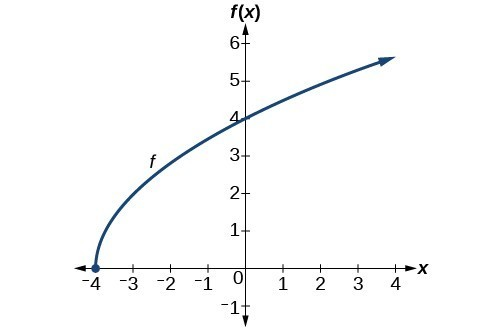Figure 20 Try It 7 Find the domain and range of $f\left(x\right)=-\sqrt{2-x}$. Solution Graphic Piecewise-Defined Functions Sometimes, we come across a function that requires more than one formula in order to obtain the given output. For example, in the toolkit functions, we introduced the absolute value function $f\left(x\right)=|x|$. With a domain of all real numbers and a range of values greater than or equal to 0, absolute value can be defined as the magnitude, or modulus, of a real number value regardless of sign. It is the distance from 0 on the number line. All of these definitions require the output to be greater than or equal to 0. If we input 0, or a positive value, the output is the same as the input. $f\left(x\right)=x\text{ if }x\ge 0$ If we input a negative value, the output is the opposite of the input. $f\left(x\right)=-x\text{ if }x<0$ Because this requires two different processes or pieces, the absolute value function is an example of a piecewise function. A piecewise function is a function in which more than one formula is used to define the output over different pieces of the domain. We use piecewise functions to describe situations in which a rule or relationship changes as the input value crosses certain “boundaries.” For example, we often encounter situations in business for which the cost per piece of a certain item is discounted once the number ordered exceeds a certain value. Tax brackets are another real-world example of piecewise functions. For example, consider a simple tax system in which incomes up to$10,000 are taxed at 10%, and any additional income is taxed at 20%. The tax on a total income, S, would be 0.1S if ${S}\le\$ $10,000 and 1000 + 0.2 (S –$10,000), if S> $10,000. A General Note: Piecewise Function A piecewise function is a function in which more than one formula is used to define the output. Each formula has its own domain, and the domain of the function is the union of all these smaller domains. We notate this idea like this: $f\left(x\right)=\begin{cases}\text{formula 1 if x is in domain 1}\\ \text{formula 2 if x is in domain 2}\\ \text{formula 3 if x is in domain 3}\end{cases}$ In piecewise notation, the absolute value function is $|x|=\begin{cases}x\text{ if }x\ge 0\\ -x\text{ if }x<0\end{cases}$ How To: Given a piecewise function, write the formula and identify the domain for each interval. 1. Identify the intervals for which different rules apply. 2. Determine formulas that describe how to calculate an output from an input in each interval. 3. Use braces and if-statements to write the function. Example 11: Writing a Piecewise Function A museum charges$5 per person for a guided tour with a group of 1 to 9 people or a fixed $50 fee for a group of 10 or more people. Write a function relating the number of people, $n$, to the cost, $C$. Solution Two different formulas will be needed. For n-values under 10, C=5n. For values of n that are 10 or greater, C=50. C(n)=$\begin{cases}{5n}\text{ if }{0}<{n}<{10}\\ 50\text{ if }{n}\ge 10\end{cases}$ Analysis of the Solution The function is represented in Figure 21. The graph is a diagonal line from $n=0$ to $n=10$ and a constant after that. In this example, the two formulas agree at the meeting point where $n=10$, but not all piecewise functions have this property.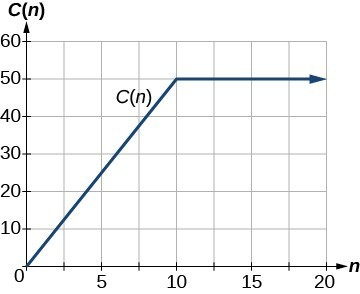Figure 21 Example 12: Working with a Piecewise Function A cell phone company uses the function below to determine the cost, $C$, in dollars for $g$ gigabytes of data transfer. $C\left(g\right)=\begin{cases}{25} \text{ if }{ 0 }<{ g }<{ 2 }\\ { 25+10 }\left(g - 2\right) \text{ if }{ g}\ge{ 2 }\end{cases}$ Find the cost of using 1.5 gigabytes of data and the cost of using 4 gigabytes of data. Solution To find the cost of using 1.5 gigabytes of data, C(1.5), we first look to see which part of the domain our input falls in. Because 1.5 is less than 2, we use the first formula. C(1.5) =$25

To find the cost of using 4 gigabytes of data, C(4), we see that our input of 4 is greater than 2, so we use the second formula.

C(4)=25 + 10( 4-2) =$45 Analysis of the Solution The function is represented in Figure 22. We can see where the function changes from a constant to a shifted and stretched identity at $g=2$. We plot the graphs for the different formulas on a common set of axes, making sure each formula is applied on its proper domain.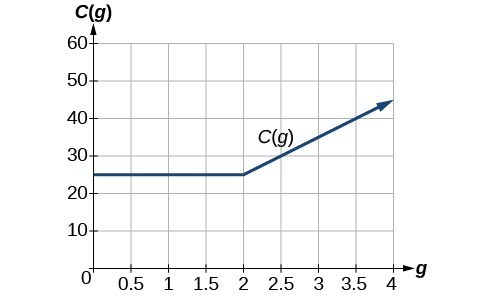Figure 22 How To: Given a piecewise function, sketch a graph. 1. Indicate on the x-axis the boundaries defined by the intervals on each piece of the domain. 2. For each piece of the domain, graph on that interval using the corresponding equation pertaining to that piece. Do not graph two functions over one interval because it would violate the criteria of a function. Example 13: Graphing a Piecewise Function Sketch a graph of the function. $f\left(x\right)=\begin{cases}{ x }^{2} \text{ if }{ x }\le{ 1 }\\ { 3 } \text{ if } { 1 }<{ x }\le 2\\ { x } \text{ if }{ x }>{ 2 }\end{cases}$ Solution Each of the component functions is from our library of toolkit functions, so we know their shapes. We can imagine graphing each function and then limiting the graph to the indicated domain. At the endpoints of the domain, we draw open circles to indicate where the endpoint is not included because of a less-than or greater-than inequality; we draw a closed circle where the endpoint is included because of a less-than-or-equal-to or greater-than-or-equal-to inequality. Below are the three components of the piecewise function graphed on separate coordinate systems.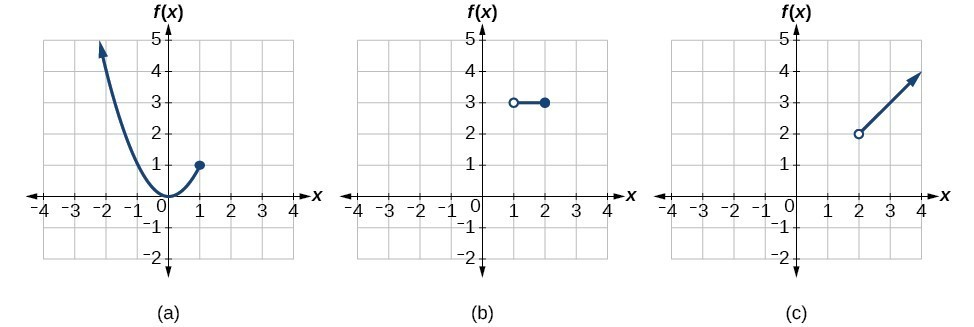(a) $f\left(x\right)={x}^{2}\text{ if }x\le 1$; (b) $f\left(x\right)=3\text{ if 1< }x\le 2$; (c) $f\left(x\right)=x\text{ if }x>2$ Now that we have sketched each piece individually, we combine them in the same coordinate plane.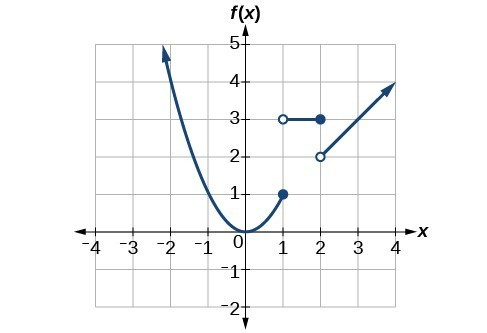Figure 24 Analysis of the Solution Note that the graph does pass the vertical line test even at $x=1$ and $x=2$ because the points $\left(1,3\right)$ and $\left(2,2\right)$ are not part of the graph of the function, though $\left(1,1\right)$ and $\left(2,3\right)$ are. Try It 8 Graph the following piecewise function. $f\left(x\right)=\begin{cases}{ x}^{3} \text{ if }{ x }<{-1 }\\ { -2 } \text{ if } { -1 }<{ x }<{ 4 }\\ \sqrt{x} \text{ if }{ x }>{ 4 }\end{cases}$ Q&A Can more than one formula from a piecewise function be applied to a value in the domain? No. Each value corresponds to one equation in a piecewise formula. Key Concepts • The domain of a function includes all real input values that would not cause us to attempt an undefined mathematical operation, such as dividing by zero or taking the square root of a negative number. • The domain of a function can be determined by listing the input values of a set of ordered pairs. • The domain of a function can also be determined by identifying the input values of a function written as an equation. • Interval values represented on a number line can be described using inequality notation, set-builder notation, and interval notation. • For many functions, the domain and range can be determined from a graph. • An understanding of toolkit functions can be used to find the domain and range of related functions. • A piecewise function is described by more than one formula. • A piecewise function can be graphed using each algebraic formula on its assigned subdomain. 1. Why does the domain differ for different functions? 2. How do we determine the domain of a function defined by an equation? 3. Explain why the domain of $f\left(x\right)=\sqrt{x}$ is different from the domain of $f\left(x\right)=\sqrt[]{x}$. 4. When describing sets of numbers using interval notation, when do you use a parenthesis and when do you use a bracket? 5. How do you graph a piecewise function? For the following exercises, find the domain of each function using interval notation. 6. $f\left(x\right)=-2x\left(x - 1\right)\left(x - 2\right)$ 7. $f\left(x\right)=5 - 2{x}^{2}$ 8. $f\left(x\right)=3\sqrt{x - 2}$ 9. $f\left(x\right)=3-\sqrt{6 - 2x}$ 10. $f\left(x\right)=\sqrt{4 - 3x}$ 11. $f\left(x\right)=\sqrt{{x}^{2}+4}$ 12. $f\left(x\right)=\sqrt{1 - 2x}$ 13. $f\left(x\right)=\sqrt{x - 1}$ 14. $f\left(x\right)=\frac{9}{x - 6} \\$ 15. $f\left(x\right)=\frac{3x+1}{4x+2}$ 16. $f\left(x\right)=\frac{\sqrt{x+4}}{x - 4}$ 17. $f\left(x\right)=\frac{x - 3}{{x}^{2}+9x - 22}$ 18. $f\left(x\right)=\frac{1}{{x}^{2}-x - 6}$ 19. $f\left(x\right)=\frac{2{x}^{3}-250}{{x}^{2}-2x - 15}$ 20. $\frac{5}{\sqrt{x - 3}}$ 21. $\frac{2x+1}{\sqrt{5-x}}$ 22. $f\left(x\right)=\frac{\sqrt{x - 4}}{\sqrt{x - 6}}$ 23. $f\left(x\right)=\frac{\sqrt{x - 6}}{\sqrt{x - 4}}$ 24. $f\left(x\right)=\frac{x}{x}$ 25. $f\left(x\right)=\frac{{x}^{2}-9x}{{x}^{2}-81}$ 26. Find the domain of the function $f\left(x\right)=\sqrt{2{x}^{3}-50x}$ by: a. using algebra. b. graphing the function in the radicand and determining intervals on the x-axis for which the radicand is nonnegative. For the following exercises, write the domain and range of each function using interval notation. 27.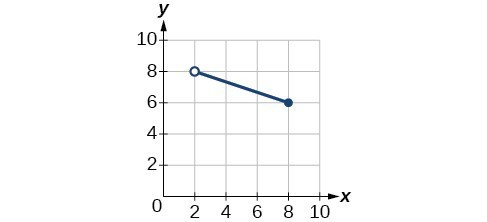Domain: ________ Range: ________ 28.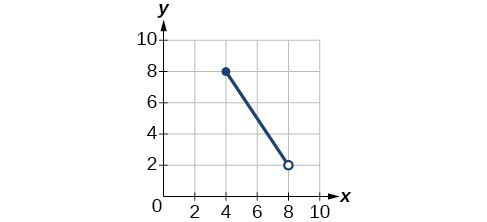29.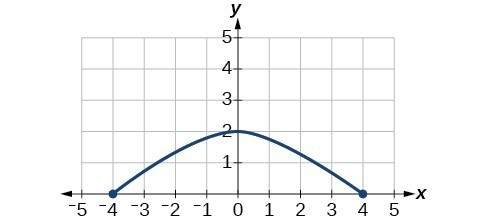30.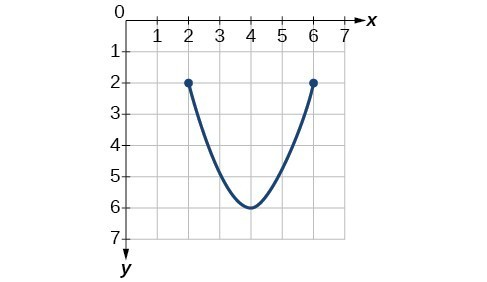31.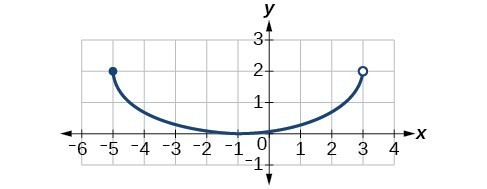32.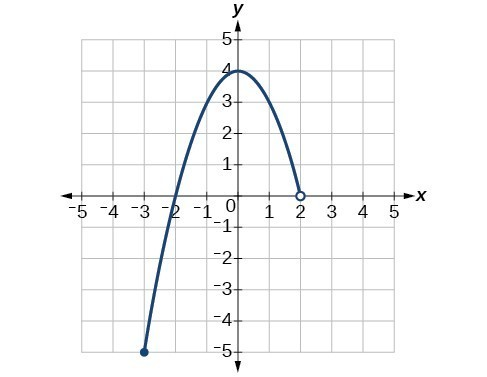33.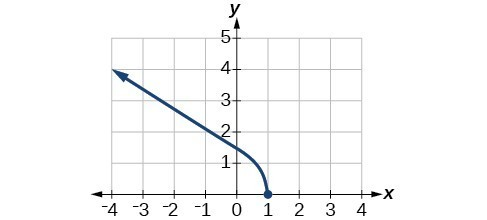34.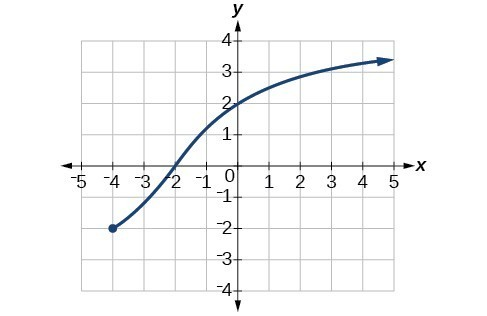35.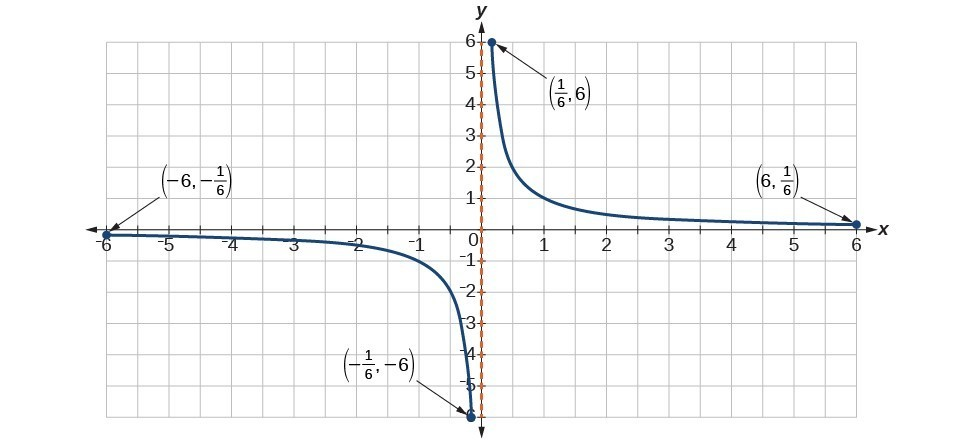36.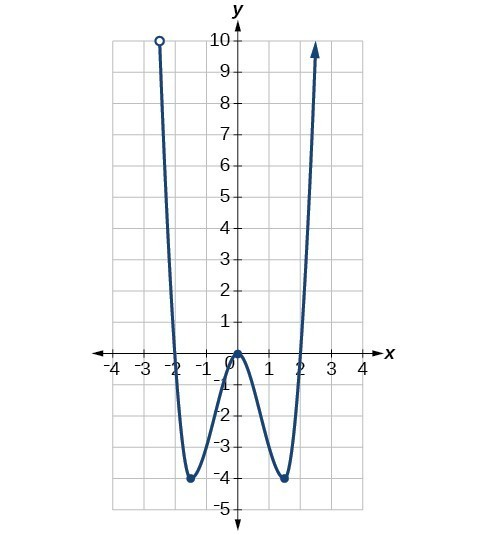37.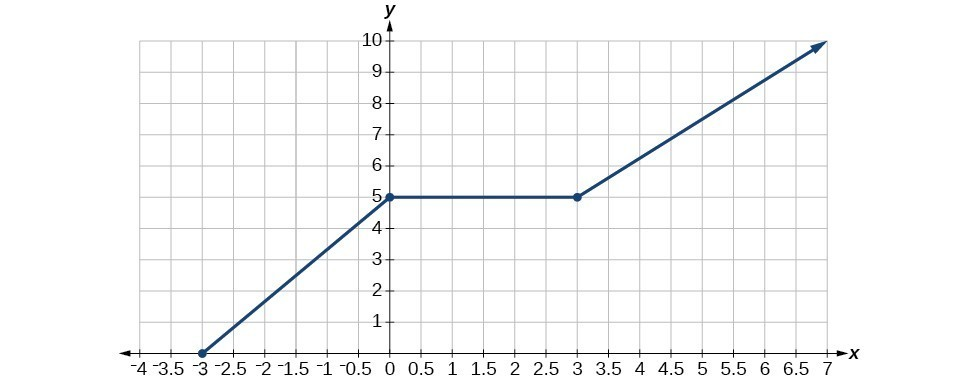For the following exercises, sketch a graph of the piecewise function. Write the domain in interval notation. 38. $f(x)=\begin{cases}{x}+{1}&\text{ if }&{ x }<{ -2 } \\{-2x - 3}&\text{ if }&{ x }\ge { -2 }\\ \end{cases}$ 39. $f\left(x\right)=\begin{cases}{2x - 1}&\text{ if }&{ x }<{ 1 }\\ {1+x }&\text{ if }&{ x }\ge{ 1 } \end{cases}$ 40. $f\left(x\right)=\begin{cases}{x+1}&\text{ if }&{ x }<{ 0 }\\ {x - 1 }&\text{ if }&{ x }>{ 0 }\end{cases}$ 41. $f\left(x\right)=\begin{cases}{3} &\text{ if }&{ x } <{ 0 }\\ \sqrt{x}&\text{ if }&{ x }\ge { 0 }\end{cases}$ 42. $f\left(x\right)=\begin{cases}{x}^{2}&\text{ if }&{ x } <{ 0 }\\ {1-x}&\text{ if }&{ x } >{ 0 }\end{cases}$ 43. $f\left(x\right)=\begin{cases}{x}^{2}&\text{ if }&{ x }<{ 0 }\\ {x+2 }&\text{ if }&{ x }\ge { 0 }\end{cases}$ 44. $f\left(x\right)=\begin{cases}x+1& \text{if}& x<1\\ {x}^{3}& \text{if}& x\ge 1\end{cases}$ 45. $f\left(x\right)=\begin{cases}|x|&\text{ if }&{ x }<{ 2 }\\ { 1 }&\text{ if }&{ x }\ge{ 2 }\end{cases}$ For the following exercises, given each function $f$, evaluate $f\left(-3\right),f\left(-2\right),f\left(-1\right)$, and $f\left(0\right)$. 46. $f\left(x\right)=\begin{cases}{ x+1 }&\text{ if }&{ x }<{ -2 }\\ { -2x - 3 }&\text{ if }&{ x }\ge{ -2 }\end{cases}$ 47. $f\left(x\right)=\begin{cases}{ 1 }&\text{ if }&{ x }\le{ -3 }\\{ 0 }&\text{ if }&{ x }>{ -3 }\end{cases}$ 48. $f\left(x\right)=\begin{cases}{-2}{x}^{2}+{ 3 }&\text{ if }&{ x }\le { -1 }\\ { 5x } - { 7 } &\text{ if }&{ x } > { -1 }\end{cases}$ For the following exercises, given each function $f$, evaluate $f\left(-1\right),f\left(0\right),f\left(2\right)$, and $f\left(4\right)$. 49. $f\left(x\right)=\begin{cases}{ 7x+3 }&\text{ if }&{ x }<{ 0 }\\{ 7x+6 }&\text{ if }&{ x }\ge{ 0 }\end{cases}$ 50. $f\left(x\right)=\begin{cases}{x}^{2}{ -2 }&\text{ if }&{ x }<{ 2 }\\{ 4+|x - 5|}&\text{ if }&{ x }\ge{ 2 }\end{cases}$ 51. $f\left(x\right)=\begin{cases}5x& \text{if}& x<0\\ 3& \text{if}& 0\le x\le 3\\ {x}^{2}& \text{if}& x>3\end{cases}$ For the following exercises, write the domain for the piecewise function in interval notation. 52. $f\left(x\right)=\begin{cases}{x+1}&\text{ if }&{ x }<{ -2 }\\{ -2x - 3}&\text{ if }&{ x }\ge{ -2 }\end{cases}$ 53. $f\left(x\right)=\begin{cases}{x}^{2}{ -2 }&\text{ if}&{ x }<{ 1 }\\{-x}^{2}+{2}&\text{ if }&{ x }>{ 1 }\end{cases}$ 54. $f\left(x\right)=\begin{cases}{ 2x - 3 }&\text{ if }&{ x }<{ 0 }\\{ -3}{x}^{2}&\text{ if }&{ x }\ge{ 2 }\end{cases}$ 55. Graph $y=\frac{1}{{x}^{2}}$ on the viewing window $\left[-0.5,-0.1\right]$ and $\left[0.1,0.5\right]$. Determine the corresponding range for the viewing window. Show the graphs. 56. Graph $y=\frac{1}{x}$ on the viewing window $\left[-0.5,-0.1\right]$ and $\left[0.1,\text{ }0.5\right]$. Determine the corresponding range for the viewing window. Show the graphs. 57. Suppose the range of a function $f$ is $\left[-5,\text{ }8\right]$. What is the range of $|f\left(x\right)|?$ 58. Create a function in which the range is all nonnegative real numbers. 59 .Create a function in which the domain is $x>2$. 60. The cost in dollars of making $x$ items is given by the function $C\left(x\right)=10x+500$. A. The fixed cost is determined when zero items are produced. Find the fixed cost for this item. B. What is the cost of making 25 items? C. Suppose the maximum cost allowed is$1500. What are the domain and range of the cost function, $C\left(x\right)?$

61. The height $h$ of a projectile is a function of the time $t$ it is in the air. The height in feet for $t$ seconds is given by the function $h\left(t\right)=-16{t}^{2}+96t$. What is the domain of the function? What does the domain mean in the context of the problem?

Glossary

interval notation
a method of describing a set that includes all numbers between a lower limit and an upper limit; the lower and upper values are listed between brackets or parentheses, a square bracket indicating inclusion in the set, and a parenthesis indicating exclusion
piecewise function
a function in which more than one formula is used to define the output
set-builder notation
a method of describing a set by a rule that all of its members obey; it takes the form $\left\{x|\text{statement about }x\right\}$